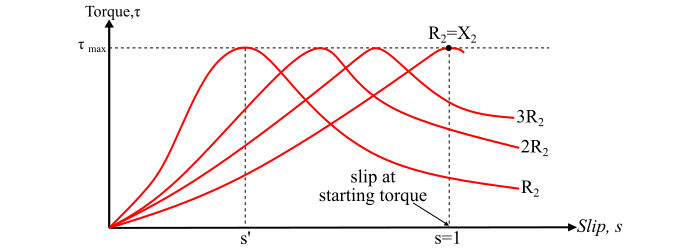# Torque Slip Characteristics of 3-Phase Induction Motor

The graph plotted between the torque and slip for a particular value of rotor resistance and reactance is known as torque-slip characteristics of the induction motor.

The torque of a 3-phase induction motor under running conditions is given by,

$$\mathrm{\tau_𝑟 =\frac{𝐾𝑠𝐸_2^2𝑅_2}{𝑅_2^2 + (𝑠𝑋_2)^2}… (1)}$$

From the eqn. (1), it can be seen that if R2 and X2 are kept constant, the torque depends upon the slip 's'. The torque-slip characteristics curve can be divided into three regions, viz.

• Low-slip region
• Medium-slip region
• High-slip region## Low-Slip Region

At synchronous speed, the slip s = 0, thus, the torque is 0. When the speed is very near to the synchronous speed, the slip is very low and the term (𝑠𝑋2)2 is negligible in comparison with R2. Therefore,

$$\mathrm{\tau_𝑟 \propto\frac{𝑠}{𝑅_2}}$$

If R2 is constant, then

$$\mathrm{\tau_𝑟 \propto 𝑠 … (2)}$$

Eqn. (2) shows that the torque is proportional to the slip. Hence, when the slip is small, the torque-slip curve is straight line.

## Medium-Slip Region

When the slip increases, the term (𝑠𝑋2)2 becomes large so that 𝑅22 may be neglected in comparison with (𝑠𝑋2)2. Therefore,

$$\mathrm{\tau_𝑟 \propto\frac{𝑠}{(𝑠𝑋_2)^2} =\frac{1}{𝑠𝑋_2^2}}$$

If X2 is constant, then

$$\mathrm{\tau_𝑟 \propto\frac{1}{𝑠}… (3)}$$

Thus, the torque is inversely proportional to slip towards standstill conditions. Hence, for intermediate values of the slip, the torque-slip characteristics is represented by a rectangular hyperbola. The curve passes through the point of maximum torque when R2 = 𝑠𝑋2.

The maximum torque developed by an induction motor is known as pull-out torque or breakdown torque. This breakdown torque is a measure of the short time overloading capability of the motor.

## High-Slip Region

The torque decreases beyond the point of maximum torque. As a result of this, the motor slows down and eventually stops. The induction motor operates for the values of slip between s = 0 and s = sm, where sm is the value of slip corresponding to maximum torque. For a typical 3-phase induction motor, the breakdown torque is 2 to 3 times of the full-load torque. Therefore, the motor can handle overloading for a short period of time without stalling.

Important – It may be seen from the torque-slip characteristics that addition of resistance to the rotor circuit does not change the value of maximum torque but it only changes the value of slip at which maximum torque occurs.